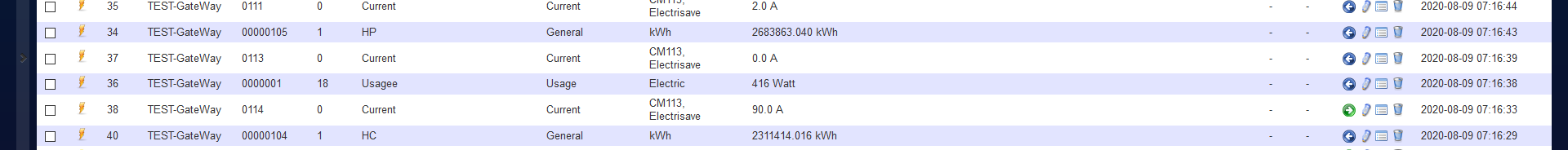# Wrong data from mysensors.

• Hi,

that's the firts time I use mysensors.
I have a device which give me the instant current and total current for my house.
In domoticz, when I go to the device screen, I can seethat for number 40 the value is 2311414.016.
But in the Utility screen, value is 0 !!! What Am I doing wrong ?Thank you.

• Hi @David-Marlinge
I have struggled with these meters in Domoticz also. I am using an S_Power meter for an energy monitor which looks like what you are using. This is my understanding:

The value on the top of the bar is the power you have most recently sent (in Watts).
Domoticz calculates kWh from the watts and the time it was sent.
kWh used today is shown on the Utility screen (your 2036.992).
Overall kWh is what is shown on the device tab (your 2311414.016).

I present an S_POWER device, then measure the current and multiply by the voltage to get the power in watts. Then I send that with a V_WATT message. I don't calculate the kWh. Domoticz calculates kWh from the Watts I send and time I send it.

For example:

``````present(CHILD_ID_PWR, S_POWER, "Emon 38/26H");
. . . . . . . .
MyMessage Msg_Pwr(CHILD_ID_POWER, V_WATT);
. . . . . .
send(Msg_Pwr.set(Pwr, 1));
``````

Then the Pwr value should show up in that top bar. Domoticz will calculate the kWh used based on the time of the power updates.
I have not really used the V_KWH variable, so I'm not sure how that fits in with this one.

5

5

3

7

4

2

4

4